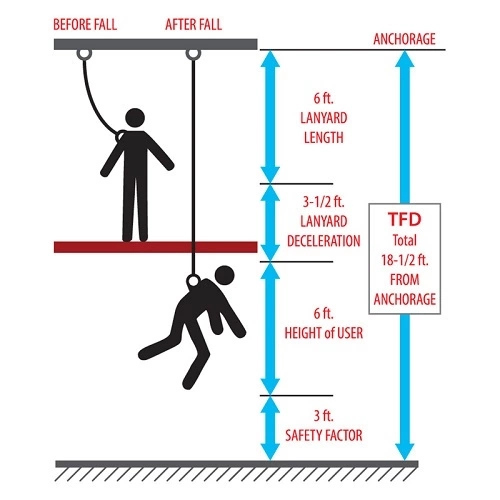# How To Calculate The Total Fall Distance Of Your LanyardThis article aims to educate us on how to calculate the total fall distance.

The total fall distance of a personal arrest system (Like your body harness) is the sum of free fall distance and deceleration distance.

According to the International Safety Equipment Association (ISEA), personal fall arrest systems must be selected and rigged to ensure that potential free fall distances will never exceed 6 ft (1.8 m) as required by OSHA. The deceleration distance and elongation that must be taken into consideration can be seen in the equipment manufacturer guide.

Also, to calculate the total fall distance, aside from the free fall distance and the deceleration distance, other parameters could be considered; like the harness effects and safety factor.

To calculate the total fall distance, we have mentioned some parameters – Free fall distance, Deceleration distance, Harness effect and Vertical elongation, safety factor to consider.

Read AlsoWhat is fall arrest system + types

Before we get into the calculation proper, let us define these parameters:

• Free fall distance: This is the vertical distance a worker travels between the onset of a fall until just prior to the point where the fall arrest system arrests the fall.
• Deceleration distance: This is the vertical distance that a worker travels between fall arrest system activation and final fall arrest.
• Harness effect: This is the stretch distance of the harness during fall. It is typically 1-ft. or fewer for a properly fitted harness.
• Vertical elongation: This is the distance of stretch of the fall arrest system lifeline.
• Safety factor: This is an additional factor to ensure that you have required clearance below your working surface.

Now that we have explained these parameters, to calculate the total fall distance will be easy.

Read AlsoWorking at height safety procedures

## To Calculate The Total Fall Distance

Fall distance is calculated by adding the values of all the above parameters.

• Free fall distance – FFD
• Deceleration distance – DD
• Vertical elongation – VE
• Harness effect -HE
• Safety factor – SF

If we use the abbreviation:

Total fall distance (TFD) = FFD + DD + VE + HE + SF

Practically:

Calculating the Total fall Distance (TFD) for a 6-foot worker using a 6-foot lanyard, the total fall distance would be 18.5 ft (5.6 m), calculated as the sum of the free fall distance (6 ft lanyard length), the deceleration distance (3.5 ft maximum allowed by OSHA for elongation of the energy absorber), harness stretch (1 ft), distance from the worker’s feet to the D-ring (5 ft) and safety factor (3 ft).

NOTE:

• Harness stretch is the harness effect
• Distance from the worker’s feet to the D-ring is the vertical elongation (VE)

You may ask, how do i know these values if am to calculate, here is how it works:

Free fall distance (FFD): If a workers anchor point is at the same vertical level with his harness back D-ring, his free fall distance will equal his lanyard length, but if the anchor point is above or below the D-ring, the FFD will change; it will not be equal to the lanyard length.

Here is the basis of the FFD calculation in that regard:

For every 1-ft. that the lanyard attachment point is above the harness back D-ring, 1-ft. is deducted from freefall distance (Harness lanyard length). For every 1-ft. that the lanyard attachment point is below the harness back D-ring, 1-ft. is added to freefall distance (harness lanyard length).

Deceleration distance: This can be extracted from the manufacturers guide.

Vertical elongation: This can just be termed elongation, it is also be gotten from the manufacturers guide.

Harness effect: This is relatively constant at less than 1-ft.

Safety factor: This factor must be at least 2ft or more.

The significance of calculating total fall distance is to help put control measures in case of fall. Sometimes it is used to estimate the distance to set an alternative platform in case of fall.

Read AlsoHow to carry out safety harness inspection and precautions

I believe the subject “How to calculate the total fall distance “has been well explained.